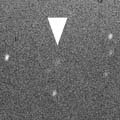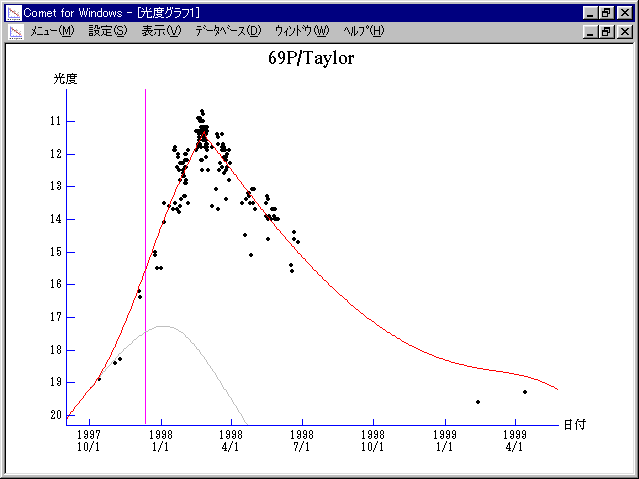# 69P/Taylor (1997)###Pictures###Orbital Elements

```   The following improved orbital elements by Kenji Muraoka,
are from 360 observations  1990 Nov. 11  to  1999 Apr. 15,
including 9 Planets Moon and 5 minor planets perturbations
and non-gravitational effect of style II.
The mean residual is +/- 0.87 arc seconds.

Epoch  =  1997 Dec. 18.0  TT       JDT = 2450800.5
T  =  1997 Dec. 12.27163       +/- 0.00318 (m.e.) TT
Peri. =  355.37636                +/- 0.00139
Node  =  108.85859                +/- 0.00003   (2000.0)
Incl. =   20.54699                +/- 0.00003
q  =    1.9478423              +/- 0.0000022 AU
e  =    0.4659871              +/- 0.0000027
a  =    3.6475567              +/- 0.0000052 AU
n  =    0.14148194             +/- 0.00000030
P  =    6.966                  +/- 0.0000148  years
A1  =   +2.238                  +/- 0.268
A2  =   +0.60905                +/- 0.05127
```

###Finding Charts1997 Sept.29 - 1998 Dec. 13

###Magnitudes Graph

```        m1 =  8.5 + 5 log d + 30   log r           [   ,-70]  (             - 1997 Oct.  3)
m1 = 40.8 + 5 log d - 78.5 log r(t + 100)  [-40, 75]  (1997 Oct.  3 - 1998 Feb. 25)
m1 =  4.8 + 5 log d + 20.0 log r           [ 75,   ]  (1998 Feb. 25 -             )

* Gray curve is:  m1 = 8.5 + 5 log d + 30 log r
```##### The orbital elements are calculated by Kenji Muraoka. The charts are made with StellaNavigator Ver.2.0 for Windows (AstroArts / ASCII). The magnitudes graphs are made with Comet for Windows.